﻿ 舰船辐射噪声的相似度评估
 舰船科学技术2019, Vol. 41Issue (3): 68-72PDF

SUN Bin, ZHENG Yuan, JIANG Bin
Navy Submarine Academy, Qingdao 266199, China
Abstract: By combing the feature extraction method of ship-radiated noise with similarity evaluation method, a evaluation system is established to evaluate the similarity degree of two groups of ship-radiated noise. In this paper, by the analysis of continuous spectrum, line spectrum and DEMON spectrum, we extract 23 features from three aspects as evaluation indicators; then through AHP and similarity degree method, determine the similarity degree and weight coefficient of similarity elements, finally establish the similarity evaluation system of ship-radiated noise. After using the evaluation method to analyze and calculate two groups of ship-radiated noise, we find the results agree well with the fact, which proves the credibility of this system and will have some reference value for the application of vessel recognition, condition monitoring and other aspects.
Key words: ship-radiated noise     feature extraction     similarity evaluation     AHP
0 引　言

1 相似度评估指标获取

1.1 连续谱指标获取

 $y = f(x,C) = {c_0} + {c_1}x + {c_2}{x^2} + {c_3}{x^3} + \cdot \cdot \cdot + {c_m}{x^m}\text{。}$ (1)

m=3，用最小二乘法计算多项式的特征参数c0c1c2c3，随后将频率轴平分为6个区间，分别提取6个区间的特征参数，对每个区间的特征参数取均值，就分别获得了6个区间的平均特征参数，它们和总的4个特征参数c0c1c2c3一起构成了连续谱的10个特征向量。

1.2 线谱指标获取

1.3 DEMON谱指标获取

DEMON谱，即调制谱。由于舰船辐射噪声中存在螺旋桨“桨拍”现象，只分析功率谱无法获得轴频等重要特征，因此需要对噪声进行DEMON分析，提取所需特征值。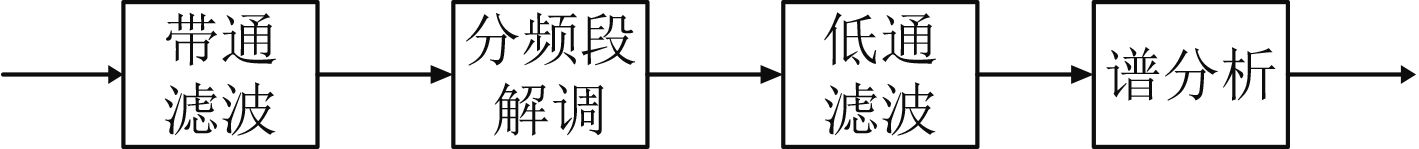图 1 DEMON谱分析流程图 Fig. 1 Flowchart of DEMON spectrum analysis

 ${D_j} = \frac{1}{N}\sum\limits_{i = 1}^N {X\left( {i \times {f_j}} \right)} \text{。}$ (2)

2 建立相似度评估体系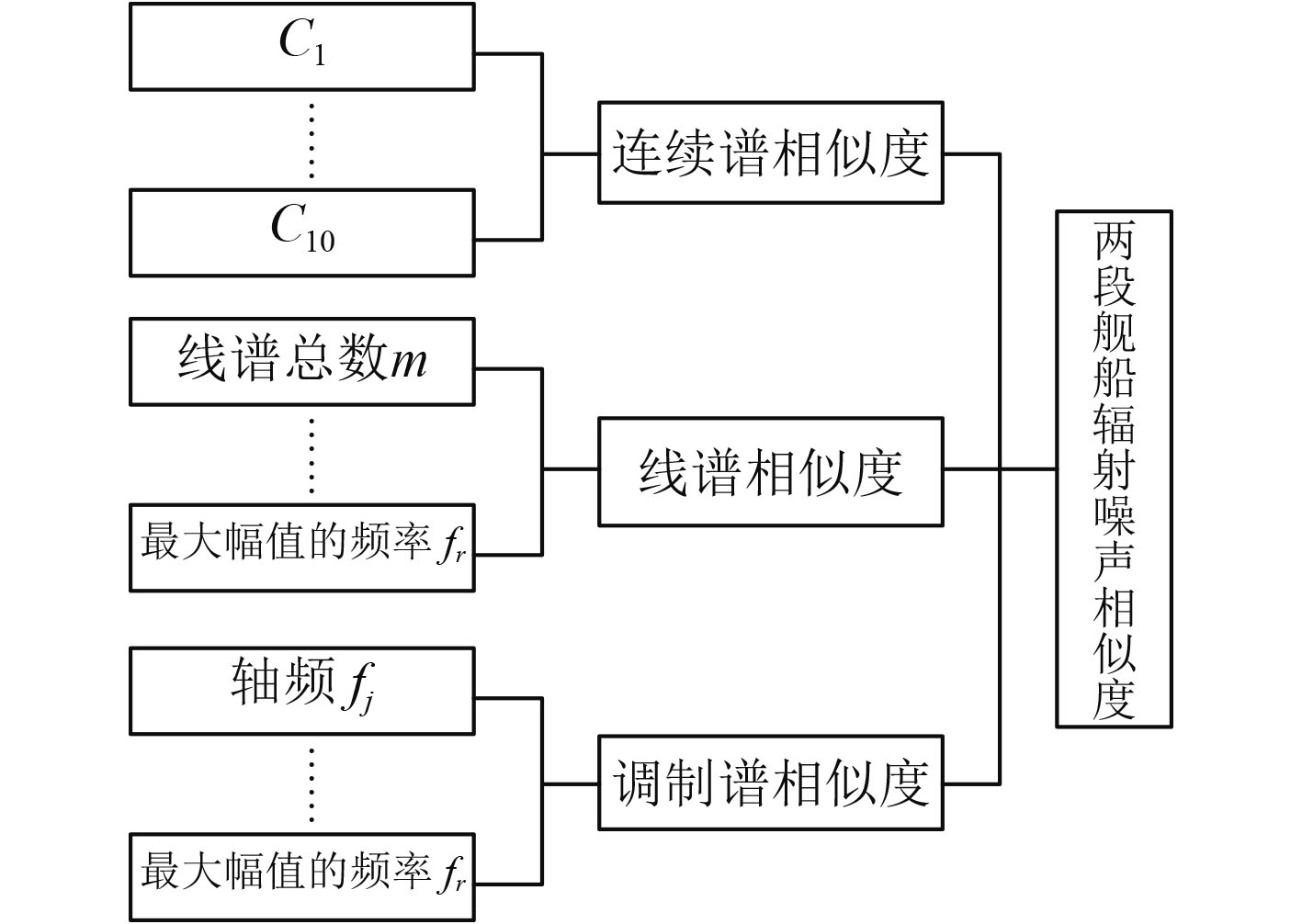图 2 相似度计算系统 Fig. 2 System diagram of similarity computation

2.1 构造相似元

2.2 计算相似元相似度

 $q({u_i}) = { {{\min ({r_i},{s_i})} \over {\max ({r_i},{s_i})}}},$ (3)

2.3 权重计算

u1u6分别为两信号mfminfwfcfafr的相似系数，则构造判断矩阵如表1所示。表 1 线谱判断矩阵 Tab.1 Judgment matrix of line spectrum

 $\begin{split} W_1 =& {\rm{[0}}{\rm{.439\;8,0}}{\rm{.270\;3,0}}{\rm{.119\;1,0}}{\rm{.071\;4,}}\\ & {\rm{0.119\;1,0}}{\rm{.043\;2]}}\text{。} \end{split}$ (4)表 2 DEMON谱判决矩阵 Tab.2 Judgment matrix of DEMON spectrum

 $\begin{split} W_2 =& {\rm{[0}}{\rm{.378,0}}{\rm{.173\;1,0}}{\rm{.173\;1,0}}{\rm{.107\;3,0}}{\rm{.066\;4,}}\\ & {\rm{0.035\;8,0}}{\rm{.066\;4]}}\text{。} \end{split}$ (5)
2.4 系统相似度计算

${{X}} = ({x_1},{x_2},{x_3}, \cdot \cdot \cdot ,{x_6})$ ${{Y}} = ({y_1},{y_2},{y_3}, \cdot \cdot \cdot ,{y_6})$ 表示2段信号提取的连续谱特征向量，采用夹角余弦法求两向量的相似度，计算公式为

 $L = sim(x,y) = \frac{{\displaystyle\sum\limits_{i = 1}^n {{x_i} \cdot {y_i}} }}{{{{\left(\displaystyle\sum\limits_{i = 1}^n {{x_i}^2 \cdot } \displaystyle\sum\limits_{i = 1}^n {{y_i}^2} \right)}^{\frac{1}{2}}}}}\text{，}$ (6)

 $Q = \sum\limits_{i = 1}^6 {W{{_u}_i}q({u_i})} \text{，}$ (7)

 $P = \sum\limits_{i = 1}^7 {W{2_i}p({u_i})} \text{。}$ (8)

u1u2u3分别代表DEMON谱、连续谱、线谱相似度，考虑到在舰船辐射噪声中，DEMON谱特征更具有代表性，构造判断矩阵如表3所示。表 3 总体判断矩阵 Tab.3 Overall judgment matrix

 $W = {\rm{[0}}{\rm{.648\;3,0}}{\rm{.122\;0,0}}{\rm{.229\;7]}}\text{，}$ (9)

 $D = {w_1}P + {w_2}L + {w_3}Q\text{。}$ (10)
3 数据处理与分析

3.1 连续谱相似度计算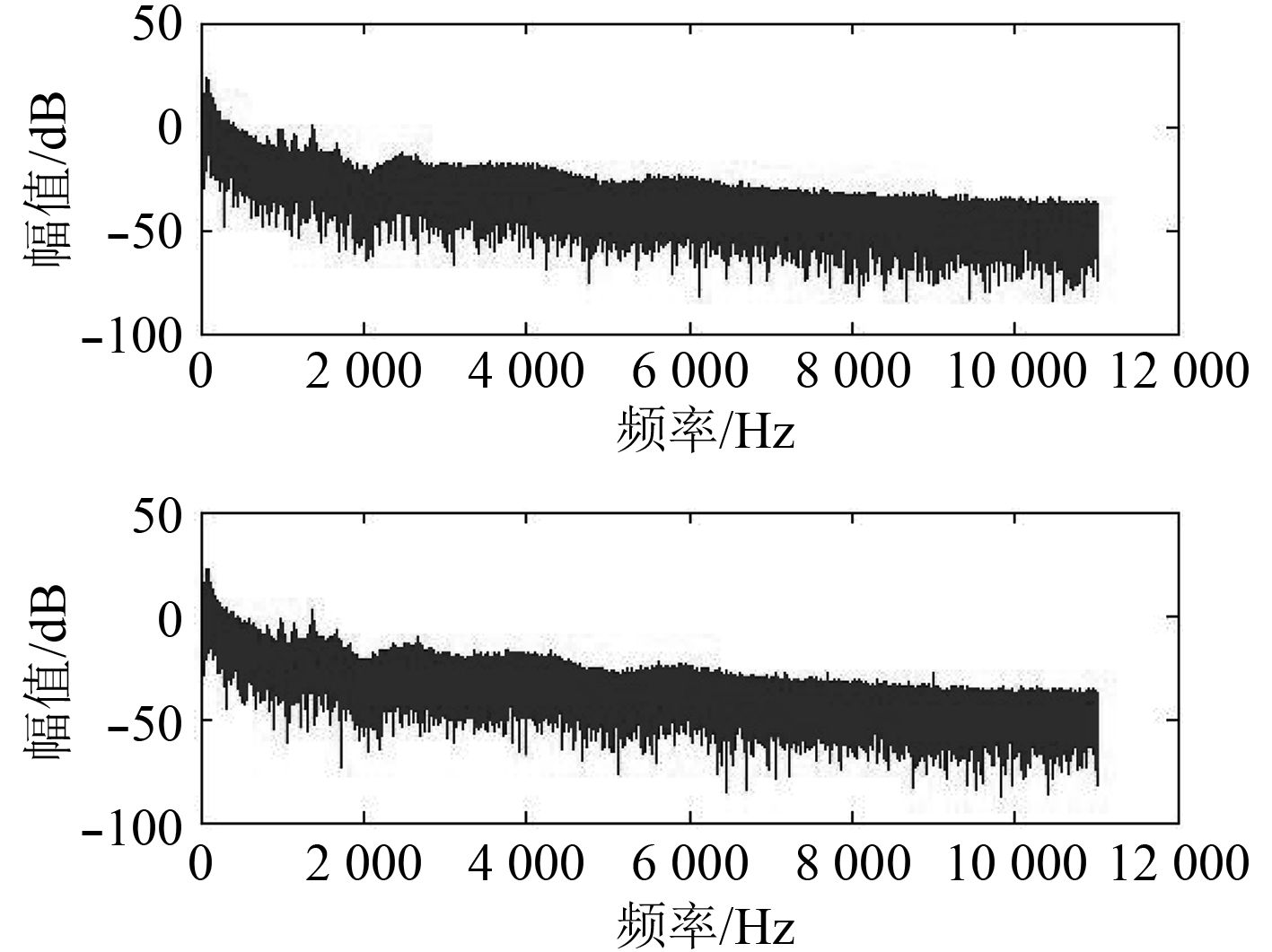图 3 辐射噪声功率谱 Fig. 3 The power spectrum of ship-radiated noise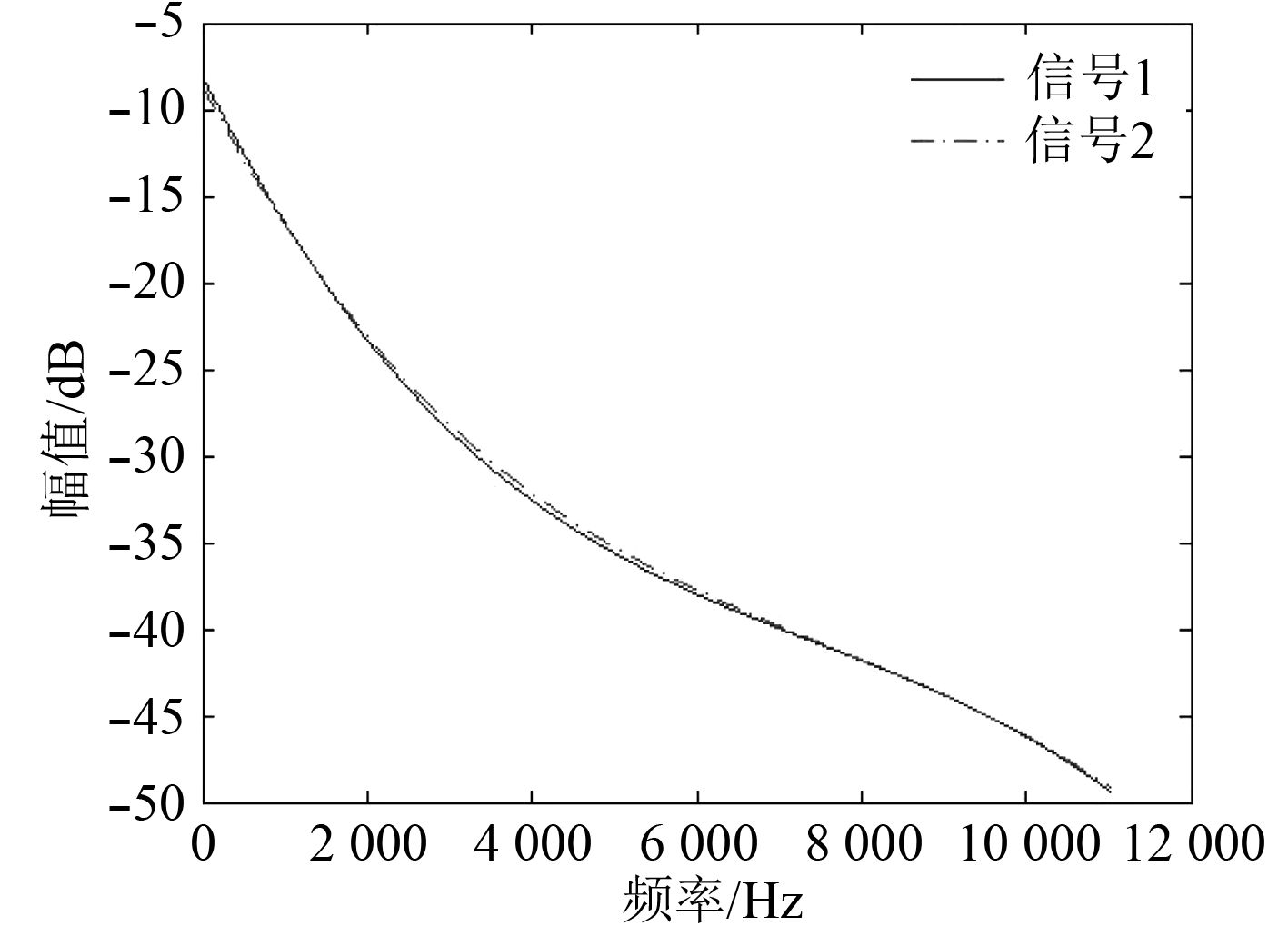图 4 连续谱趋势项 Fig. 4 Continuous spectrum trend

X=[0.000 0, –0.009 5, –8.060 3, –0.103 8, –48.433 1, –180.278 7, –244.656 1, –5.741 8, 7.371 1]，

Y=[0.000 0, –0.008 9, –8.65, –0.486 2, –55.172 3, –196.853 6, –292.887 6, –119.102 2, 65.765 4]。

3.2 线谱相似度计算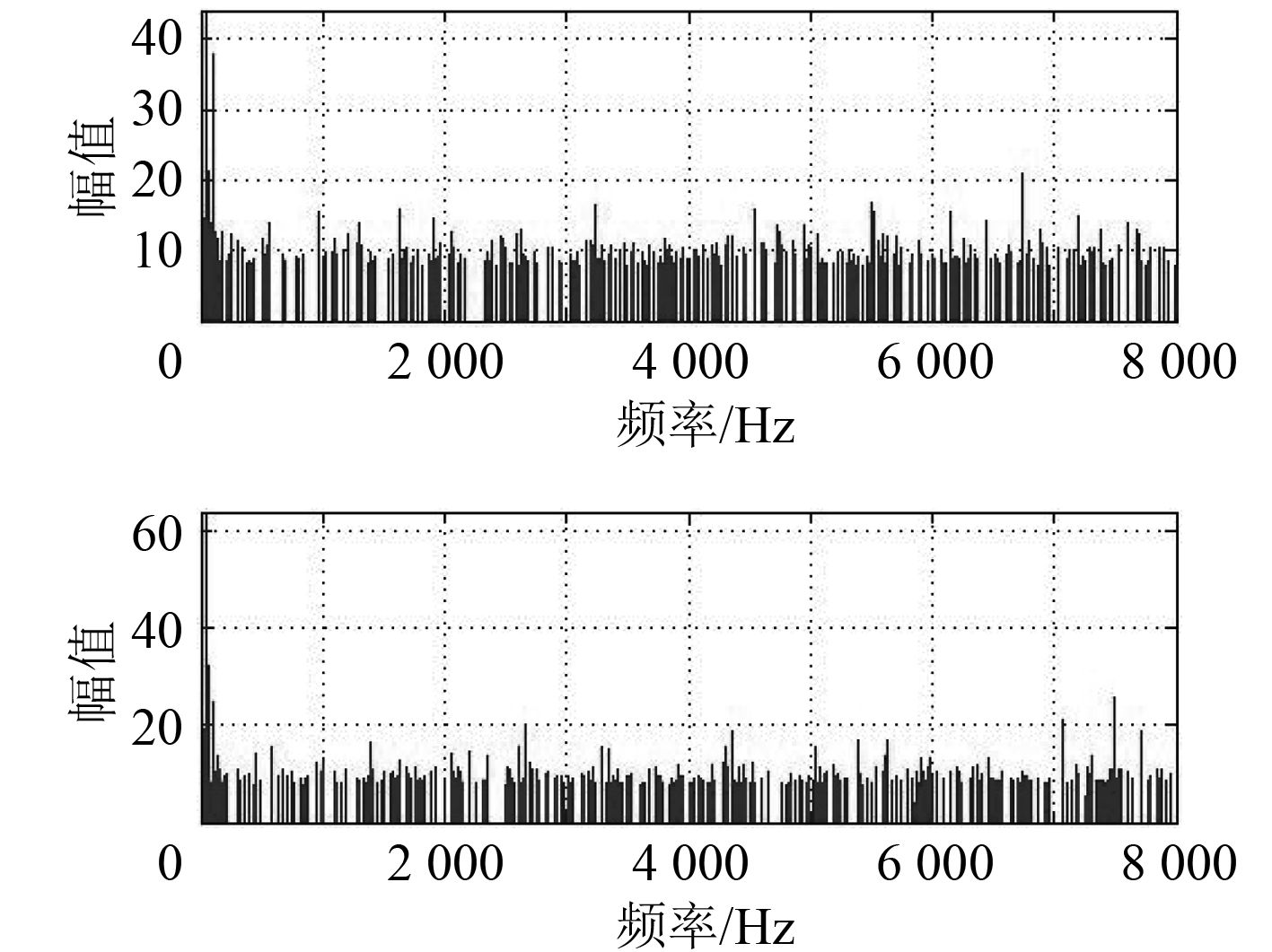图 5 提取的线谱 Fig. 5 Extracted line spectrum

S=[488, 24, 10 991, 5 660, 23, 48]；

R=[471, 24, 10 984, 5 471, 23, 48]。

 $q({u_i}) = {\rm{[0}}{\rm{.965\;2,1}}{\rm{.000\;0,0}}{\rm{.999\;4,0}}{\rm{.966\;5,0}}{\rm{.965\;8,1}}{\rm{.000\;0]}},$

3.3 DEMON谱相似度计算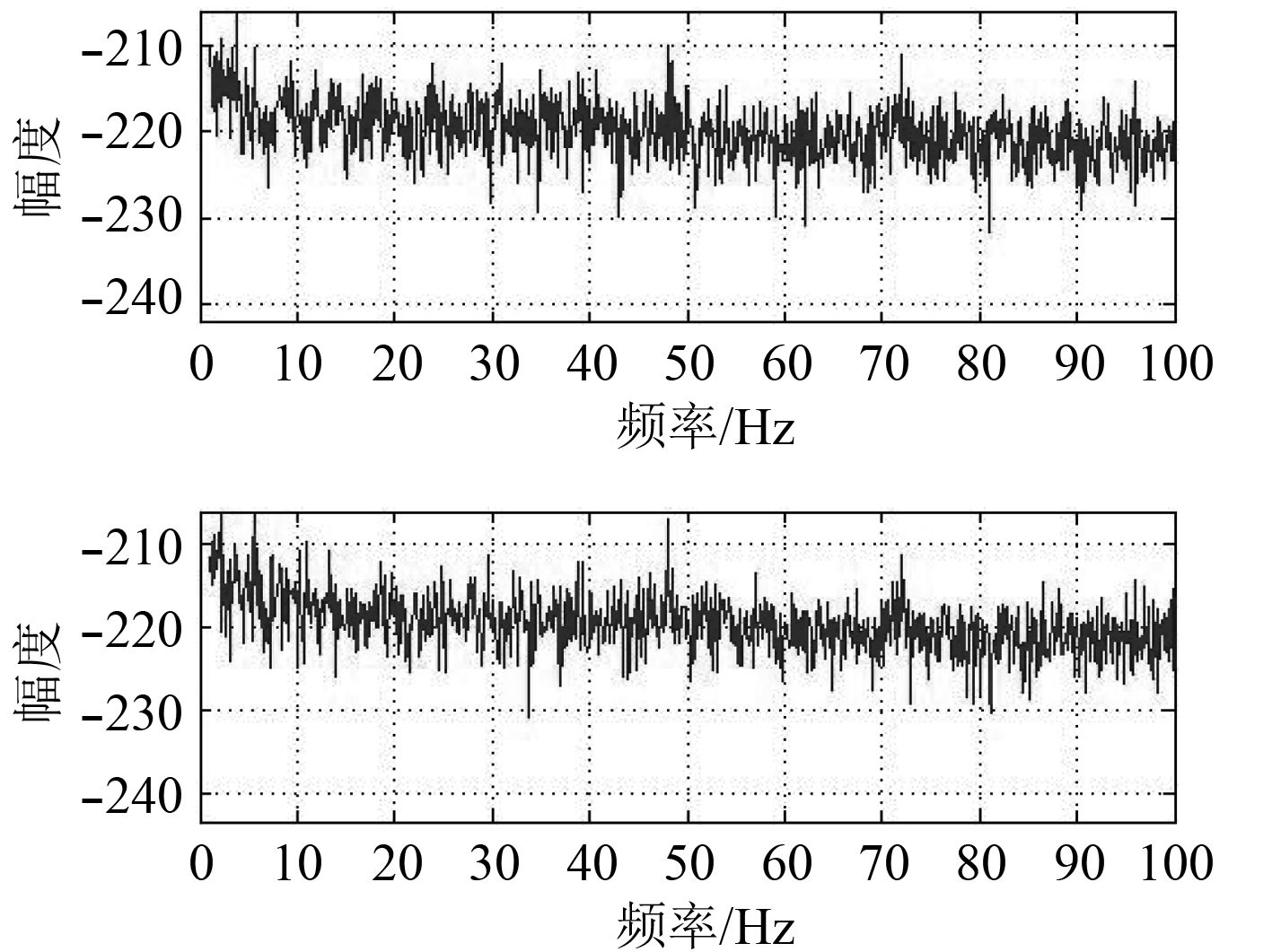图 6 DEMON谱 Fig. 6 DEMON spectrum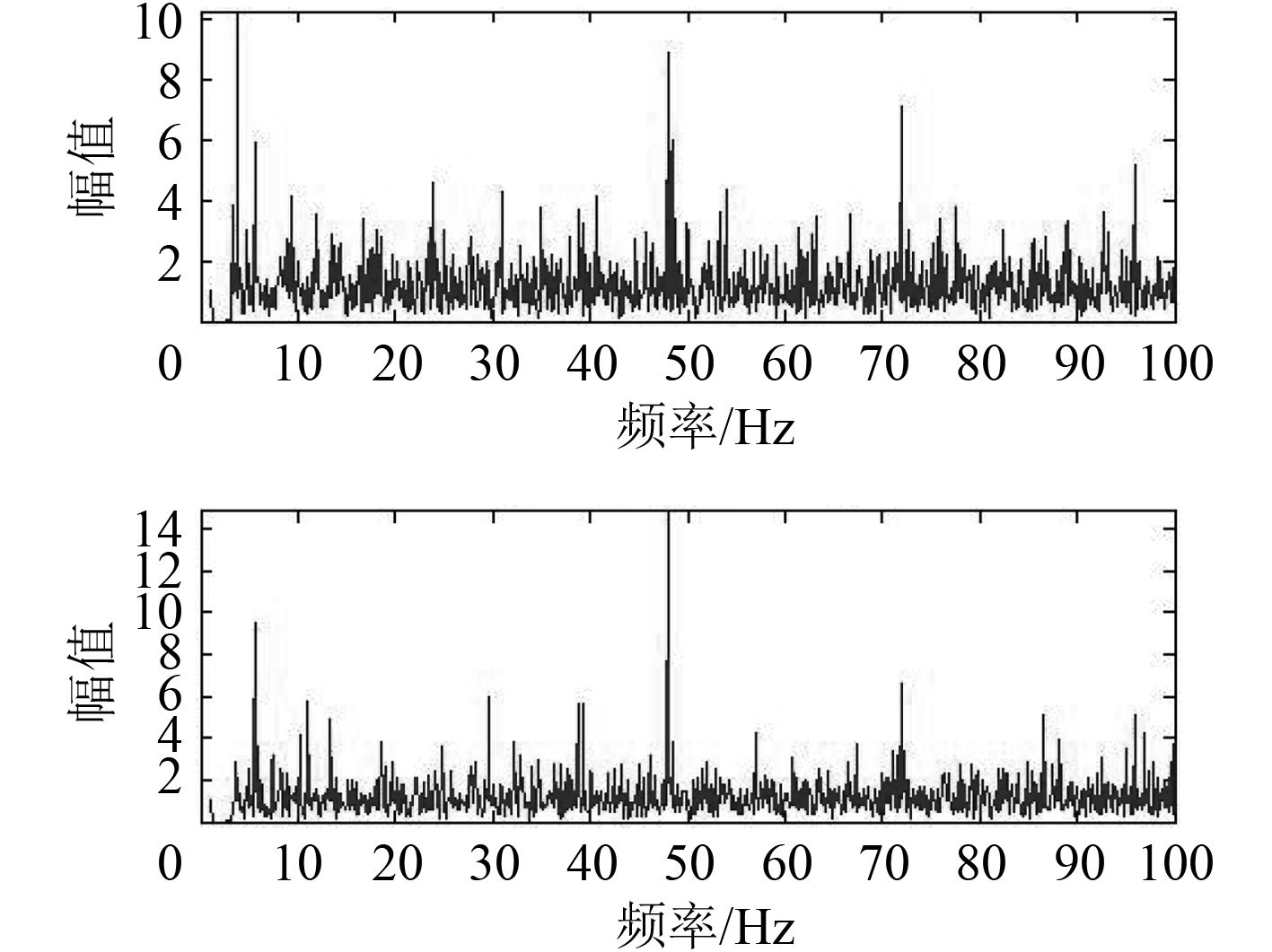图 7 拉平的DEMON谱 Fig. 7 Flattened DEMON spectrum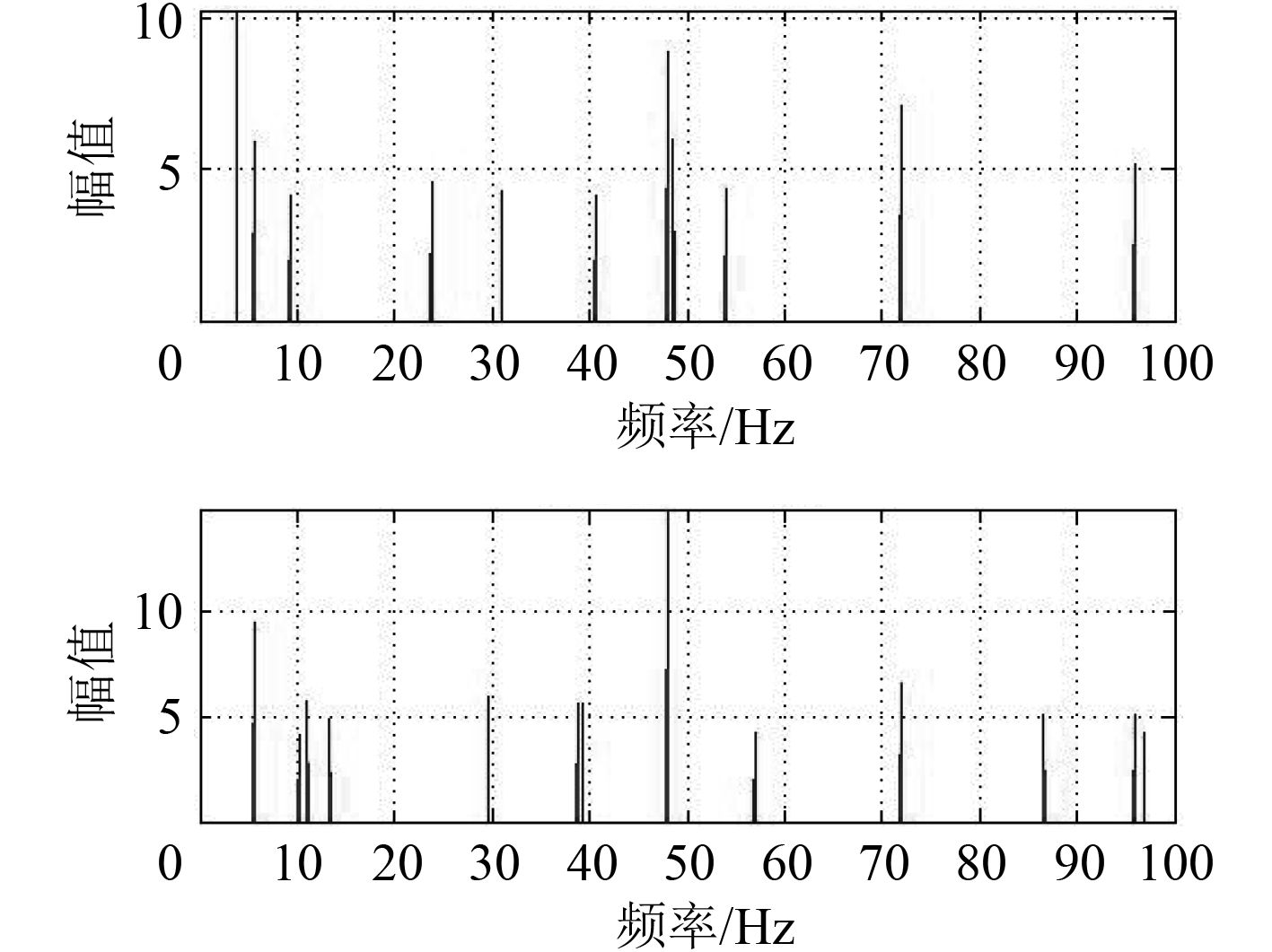图 8 DEMON线谱 Fig. 8 DEMON line spectrum

M=[5.6，23，3.8，266.398 8，108.257 1，11.582 6，3.8]，

N=[5.6，20，5.6，236.398 9，82，213 3，11.819 9，47.999 8]，

 $\begin{split}{l} &p({u_i}) = {\rm{[1,0}}{\rm{.869\;6,0}}{\rm{.678\;6,0}}{\rm{.887\;4,0}}{\rm{.759\;4,0}}{\rm{.977\;9,}}\!\!\!\!\! \\ &\!{\rm{0.079\;2]}}\text{。} \end{split}$

3.4 总相似度计算

3个子系统的相似度L=0.942 3，Q=0.978 1，P=0.831 9，根据式（9）和式（10）得两信号的相似度D=0.875 3。表 4 船舶1在两段时间的辐射噪声相似度 Tab.4 Radiated noise similarity degree of vessel 1 in twice time表 5 船舶2与船舶1的噪声相似度 Tab.5 Radiated noise similarity degree between vessel 1 and vessel 2

4 结　语

  D. 罗斯. 水下噪声原理[M].北京: 海洋出版社, 1983.  曾庆军, 王菲, 黄国建. 基于连续谱特征提取的被动声纳目标识别技术[J]. 上海交通大学学报, 2002, 36(3): 382-386. DOI:10.3321/j.issn:1006-2467.2002.03.023  单广超, 赵汉波. 舰船辐射噪声线谱检测与分析[J]. 舰船电子工程, 2014, 10: 119-112.  孙焱. 被动目标分频段调制特征提取方法研究[D].哈尔滨: 哈尔滨工程大学, 2007.  王洪玲. 舰船辐射噪声调制特征提取方法研究[D].哈尔滨: 哈尔滨工程大学, 2007.  徐迪. 基于相似理论的系统仿真方法[J]. 系统工程理论与实践, 2011, 2: 113-116. DOI:10.12011/1000-6788(2011)1-113  周美立. 相似性科学[M].北京: 科学出版社, 2004.  鹿亮. 发动机轴承仿真系统可信度评估方法和系统实现[D]. 大连: 大连理工大学, 2013.  张宇, 刘东, 计钊. 向量相似度测度方法[J]. 声学技术, 2009, 28(4): 532-536. DOI:10.3969/j.issn1000-3630.2009.04.021